# vs.eyeandcontacts.com

## Chapter 6 Integers Exercise 6.1

Question 1: Write opposites of the following:
a) Increase in weight
b) 30 km north
c) 80 m east
d) Loss of Rs 700
e) 100 m above sea level

a) Decrease in weight
b) 30 km south
c) 80 m west
d) Profit of Rs 700
d) 100 m below sea level

Question 2: Represent the following numbers as integers with appropriate signs.
a) An aeroplane is flying at a height two thousand metre above the ground.
b) A submarine is moving at a depth, eight hundred metre below the sea level.
c) A deposit of rupees two hundred.
d) Withdrawal of rupees seven hundred.

a) +2000
b) -800
c) +200
d) -700

Question 3: Represent the following numbers on a number line:
a) +5
b) -10
c) +8
d) -1
e) -6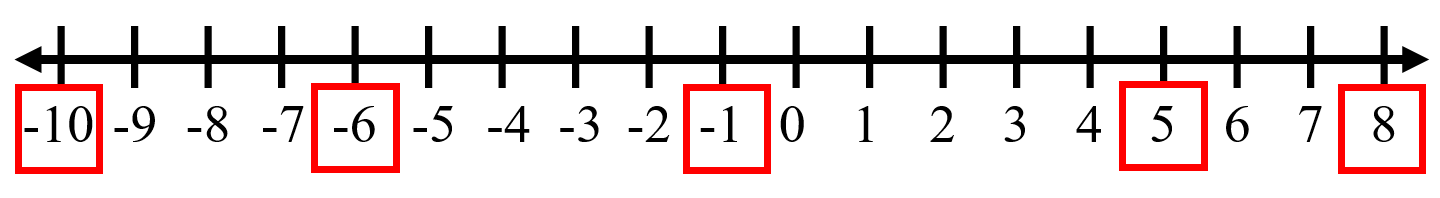Question 4: Adjacent figure is a vertical number line, representing integers. Observe it and locate the following points: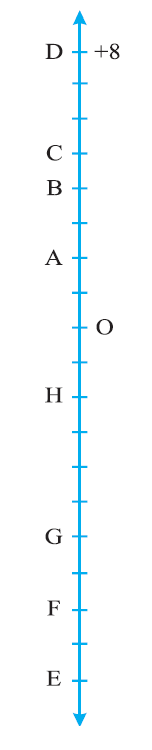a) If point D is + 8, then which point is -8?
b) Is point G a negative integer or a positive integer?
c) Write integers for points B and E.
d) Which point marked on this number line has the least value?
e) Arrange all the points in decreasing order of value.

a) F is -8.
b) Negative integer
c) B → +4, E → -10
d) E has the least value.
e) D, C, B, A, O, H, G, F, E

Question 5: Following is the list of temperatures of five places in India on a particular day of the year.a) Write the temperatures of these places in the form of integers in the blank column.
b) Following is the number line representing the temperature in degree Celsius. Plot the name of the city against its temperature.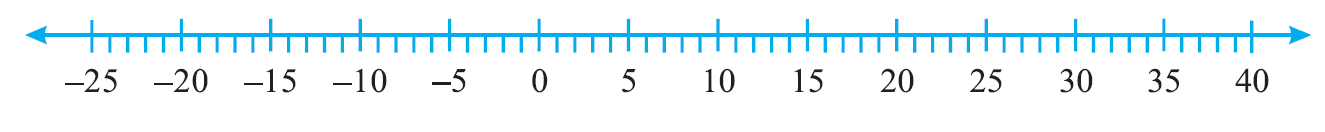c) Which is the coolest place?
d) Write the names of the places where temperatures are above 10°C.

a)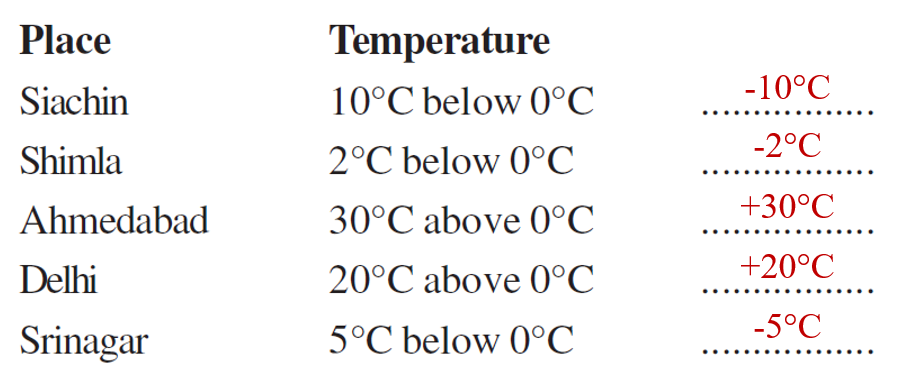b)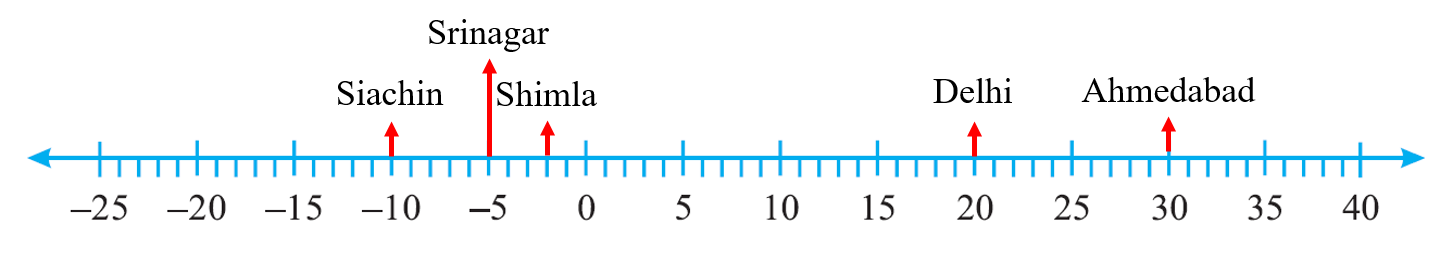c) Siachin is the coolest place.

d) Ahmedabad and Delhi are the 2 places where the temperature is above 10°C.

Question 6: In each of the following pairs, which number is to the right of the other on the number line?
a) 2, 9
b) -3, -8
c) 0, -1
d) -11, 10
e) -6, 6
f) 1, -100

a) 9 appears to the right of 2 on the number line.
b) -3 appears to the right of -8 on the number line.
c) 0 appears to the right of -1 on the number line.
d) 10 appears to the right of -11 on the number line.
e) 6 appears to the right of -6 on the number line.
f) 1 appears to the right of -100 on the number line.

Question 7: Write all the integers between the given pairs (write them in the increasing order.)
a) 0 and -7
b) -4 and 4
c) -8 and -15
d) -30 and -23

a) -6, -5, -4, -3, -2, -1
b) -3, -2, -1, 0, 1, 2, 3
c) -14, -13, -12, -11, -10, -9
d) -29, -28, -27, -26, -25, -24

Question 8:
a) Write four negative integers greater than -20.
b) Write four integers less than -10.

a) -18, 0, 10, 105
b) -21, -50, -89, -100

Question 9: For the following statements, write True (T) or False (F). If the statement is false, correct the statement.
a) -8 is to the right of -10 on a number line.
b) -100 is to the right of -50 on a number line.
c) Smallest negative integer is -1.
d) -26 is greater than -25.